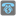• # Don't understand how calc seconds.

## Question related to missionCall to Home

If there were two calls in day, for example - 4500 and 1800, How do calc bill? 4500/60 + 1500/60 + (1800-1500)/60= 75 + 25 + 5*2 = 110, is it correct?

My code:

```def total_cost(bills):
bill_dict={}
for days in bills:
days=days.split(" ")
if days in bill_dict.keys():
bill_dict[days].append(days)
else:
bill_dict[days]=[days]
print(bill_dict)
for key in bill_dict.keys():
money_day=0
duration_day=0
for duration in bill_dict[key]:
if duration_day+int(duration) <=6000:
money_day+=int(duration)//60 + min(1,int(duration)%60)
# print(money_day)
if duration_day + int(duration) > 6000:
cache=max(0,6000 - duration_day)
money_day+=cache//60
money_day+=(int(duration)-cache)//60 * 2 + 2*min(1,(int(duration)-cache)%60)

#print(int(duration),money_day,cache)
duration_day+=int(duration)
print(key,duration_day,bill_dict[key], money_day)

bill_dict[key].append(money_day)